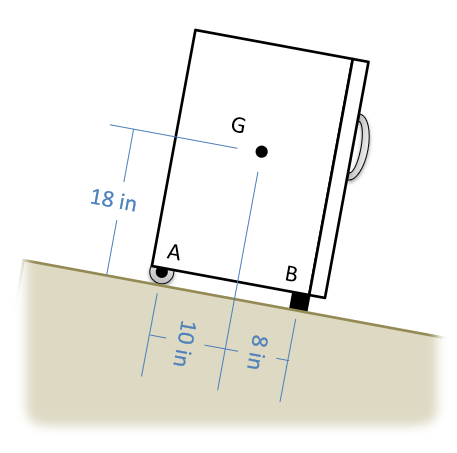﻿ Mechanics Map - Dry Friction

# Dry Friction

Dry friction is the force that opposes one solid surface sliding across another solid surface. Dry friction always opposes the surfaces sliding relative to one another and can have the effect of either opposing motion or causing motion in bodies.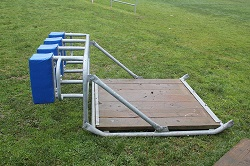Dry friction occurs between the bottom of this training sled and the grassy field. The dry friction would oppose the motion of the sled along the field in this case. Image by Avenue CC-BY-SA 3.0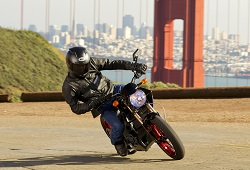Dry friction occurs between the tires and the road for this motorcycle. The dry friction force for this motorcycle is what allows it to accelerate, deccelerate, and turn. Public Domain image by Takisha Rappold.

The most commonly used model for dry friction is coulomb friction. This type of friction can further be broken down into static friction and kinetic friction. These two types of friction are illustrated in the diagram below. First imagine a box sitting on a surface. A pushing force is applied parallel to the surface and is constantly being increased. A gravitational force, a normal force, and a frictional force are also acting on the box.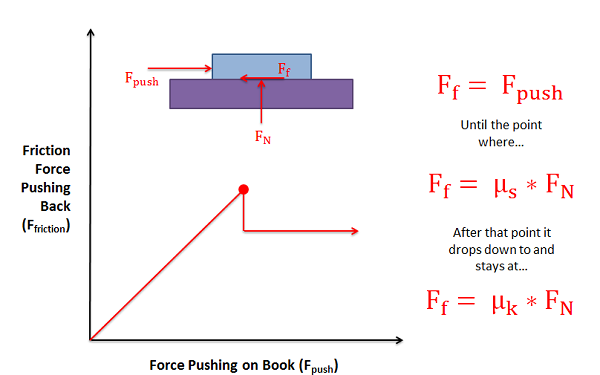As the pushing force increases, the static friction force will be equal in magnitude and opposite in directionuntil the point of impending motion. Beyond this point, the box will begin to slip as the pushing force is greater in magnitude than the kinetic friction force opposing it.

Static friction occurs prior to the box slipping and moving. In this region the friction force will be equal in magnitude and opposite in direction to the pushing force itself. As the magnitude of the pushing force increases so does the magnitude of the friction force.

If the magnitude of the pushing force continues to rise, eventually the box will begin to slip. As the box begins to slip the type of friction opposing the motion of the box changes from static friction to what is called kinetic friction. The point just before the box slips is known as impending motion. This can also be thought of as the maximum static friction force before slipping. The magnitude of the maximum static friction force is equal to the static coefficient of friction times the normal force existing between the box and the surface. This coefficient of friction is a property that depends on both materials and can usually be looked up in tables.

Kinetic friction occurs beyond the point of impending motion when the box is sliding. With kinetic friction, the magnitude of the friction force opposing motion will be equal to the kinetic coefficient of friction times the normal force between the box and the surface. The kinetic coefficient of friction also depends upon the two materials in contact, but will almost always be less than the static coefficient of friction.

## Worked Problems:

### Question 1:

A 500 lb box is sitting on concrete floor. If the static coefficient of friction is .7 and the kinetic coefficient of friction is .6:

• What is the friction force if the pulling force is 150 lbs?
• What pulling force would be required to get the box moving?
• What is the minimum force required to keep the box moving once it has started moving?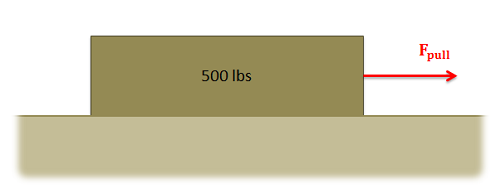### Question 2:

A 30 lb sled is being pulled up an icy incline of 25 degrees. If the static coefficient of friction between the ice and the sled is .4 and the kinetic coefficient of friction is .3, what is the required pulling force needed to keep the sled moving at a constant rate?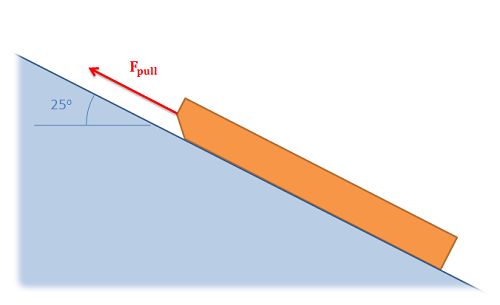### Question 3:

A plastic box is sitting on a steel beam. One end of the steel beam is slowly raised, increasing the angle of the surface until the box begins to slip. If the box begins to slip when the beam is at an angle of 41 degrees, what is the static coefficient of friction between the steel beam and the plastic box?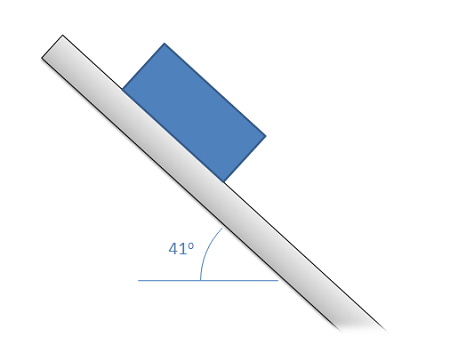### Question 4:

A 60 kg wheelbarrow with the dimensions shown below is subjected to a pulling force. If there is assumed to be no friction at the wheel at B, and the static coefficient of friction at A is assumed to be .4, what is the expected pulling force needed to get the wheelbarrow moving?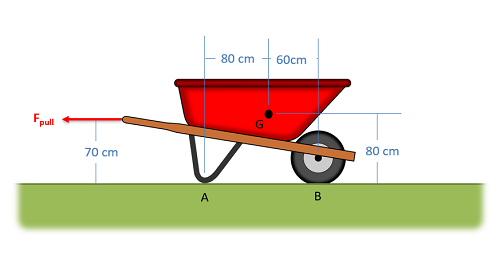### Question 5:

A cabinet with a weight of 30 lbs is sitting on a 15 degree incline as shown below. The cabinet has a pair of rubber feet at the front (B), and a pair of frictionless wheels at the back (A). What is the minimum value for the static coefficient of friction for the rubber feet at the front to ensure the cabinet does not roll down the incline?